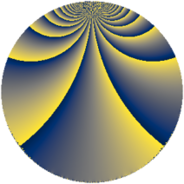# Properties

 Label 784.4.mLevel $784$ Weight $4$ Character orbit 784.m Rep. character $\chi_{784}(197,\cdot)$ Character field $\Q(\zeta_{4})$ Dimension $482$ Sturm bound $448$

# Related objects

## Defining parameters

 Level: $$N$$ $$=$$ $$784 = 2^{4} \cdot 7^{2}$$ Weight: $$k$$ $$=$$ $$4$$ Character orbit: $$[\chi]$$ $$=$$ 784.m (of order $$4$$ and degree $$2$$) Character conductor: $$\operatorname{cond}(\chi)$$ $$=$$ $$16$$ Character field: $$\Q(i)$$ Sturm bound: $$448$$

## Dimensions

The following table gives the dimensions of various subspaces of $$M_{4}(784, [\chi])$$.

Total New Old
Modular forms 688 502 186
Cusp forms 656 482 174
Eisenstein series 32 20 12

## Trace form

 $$482q + 2q^{2} + 2q^{3} - 8q^{4} + 2q^{5} - 28q^{6} + 32q^{8} + O(q^{10})$$ $$482q + 2q^{2} + 2q^{3} - 8q^{4} + 2q^{5} - 28q^{6} + 32q^{8} - 52q^{10} - 18q^{11} + 104q^{12} + 2q^{13} - 140q^{15} - 16q^{16} + 4q^{17} - 78q^{18} - 22q^{19} - 112q^{20} + 144q^{22} - 344q^{24} - 260q^{26} + 80q^{27} + 190q^{29} + 112q^{30} - 368q^{31} - 8q^{32} + 4q^{33} + 760q^{34} - 1300q^{36} + 10q^{37} - 1008q^{38} + 516q^{40} - 38q^{43} - 1400q^{44} - 194q^{45} + 500q^{46} + 944q^{47} + 676q^{48} + 2814q^{50} - 312q^{51} + 2972q^{52} + 378q^{53} + 512q^{54} + 1180q^{58} + 334q^{59} - 2860q^{60} + 914q^{61} - 412q^{62} - 1064q^{64} - 484q^{65} - 5328q^{66} - 1942q^{67} - 2640q^{68} + 476q^{69} - 36q^{72} + 2352q^{74} - 2558q^{75} + 1836q^{76} - 3280q^{78} - 4408q^{79} + 1228q^{80} - 32558q^{81} + 2748q^{82} - 118q^{83} + 480q^{85} - 9460q^{86} - 2796q^{88} - 4832q^{90} + 732q^{92} - 2404q^{93} + 3628q^{94} - 820q^{95} - 2344q^{96} + 4q^{97} + 342q^{99} + O(q^{100})$$

## Decomposition of $$S_{4}^{\mathrm{new}}(784, [\chi])$$ into newform subspaces

The newforms in this space have not yet been added to the LMFDB.

## Decomposition of $$S_{4}^{\mathrm{old}}(784, [\chi])$$ into lower level spaces

$$S_{4}^{\mathrm{old}}(784, [\chi]) \cong$$ $$S_{4}^{\mathrm{new}}(16, [\chi])$$$$^{\oplus 3}$$$$\oplus$$$$S_{4}^{\mathrm{new}}(112, [\chi])$$$$^{\oplus 2}$$